# waynehu

## Professor, Department of Astronomy and Astrophysics University of Chicago

Group Contact CV SnapShots

## Mapmaking

An experiment can be characterized by the data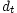taken at many different times; a pointing matrix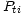, relating the data timestream to the underlying signal at pixelized positions indexed by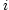, and a noise matrix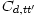characterizing the covariance of the noise in the timestream. A model for the data then is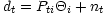(with implicit sum over the repeating index); it is the sum of signal plus noise. Here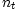is drawn from a distribution (often Gaussian) with mean zero and covariance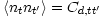. In its simplest form the pointing matrix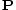contains rows - which corresponds to a particular time - with all zeroes in it except for one column with a one (see Figure 5). That column corresponds to the particular pixel observed at the time of interest. Typically, a pixel will be scanned many times during an experiment, so a given column will have many ones in it, corresponding to the many times the pixel has been observed.

Given this model, a well-posed question is: what is the optimal estimator for the signal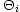? i.e. what is the best way to construct a map? The answer stems from the likelihood function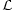, defined as the probability of getting the data given the theory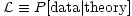. In this case, the theory is the set of parameters,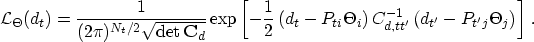(26)

That is, the noise, the difference between the data and the modulated signal, is assumed to be Gaussian with covariance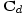.

There are two important theorems useful in the construction of a map and more generally in each step of the data pipeline [Tegmark et al, 1997]. The first is Bayes' Theorem. In this context, it says that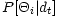, the probability that the temperatures are equal togiven the data, is proportional to the likelihood function times a prior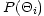. Thus, with a uniform prior,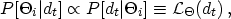(27)

with the normalization constant determined by requiring the integral of the probability over allto be equal to one. The probability on the left is the one of interest. The most likely values oftherefore are those which maximize the likelihood function. Since the log of the likelihood function in question, Equation (26), is quadratic in the parameters, it is straightforward to find this maximum point. Differentiating the argument of the exponential with respect toand setting to zero leads immediately to the estimator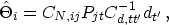(28)

where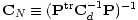. As the notation suggests, the mean of the estimator is equal to the actualand the variance is equal to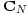.

The second theorem states that this maximum likelihood estimator is also the minimum variance estimator. The Cramer-Rao inequality says no estimator can measure thewith errors smaller than the diagonal elements of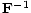, where the Fisher matrix is defined as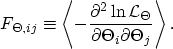(29)

Inspection of Equation (26) shows that, in this case the Fisher matrix is precisely equal to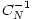. Therefore, the Cramer-Rao theorem implies that the estimator of Equation (28) is optimal: it has the smallest possible variance [Tegmark, 1997a]. No information is lost if the map is used in subsequent analysis instead of the timestream data, but huge factors of compression have been gained. For example, in the recent Boomerang experiment [Netterfield et al, 2001], the timestream containednumbers, while the map had only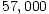pixels. The map resulted in compression by a factor of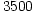.

There are numerous complications that must be dealt with in realistic applications of Equation (28). Perhaps the most difficult is estimation of, the timestream noise covariance. This typically must be done from the data itself [Ferreira & Jaffe, 2000,Stompor et al, 2001]. Even ifwere known perfectly, evaluation of the map involves inverting, a process which scales as the number of raw data points cubed. For both of these problems, the assumed stationarity of(it depends only on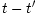) is of considerable utility. Iterative techniques to approximate matrix inversion can also assist in this process [Wright et al, 1996]. Another issue which has received much attention is the choice of pixelization. The community has converged on the Healpix pixelization scheme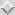, now freely available.

Perhaps the most dangerous complication arises from astrophysical foregrounds, both within and from outside the Galaxy, the main ones being synchrotron, bremmsstrahlung, dust and point source emission. All of the main foregrounds have different spectral shapes than the blackbody shape of the CMB. Modern experiments typically observe at several different frequencies, so a well-posed question is: how can we best extract the CMB signal from the different frequency channels [Bouchet & Gispert, 1999]? The blackbody shape of the CMB relates the signal in all the channels, leaving one free parameter. Similarly, if the foreground shapes are known, each foreground comes with just one free parameter per pixel. A likelihood function for the data can again be written down and the best estimator for the CMB amplitude determined analytically. While in the absence of foregrounds, one would extract the CMB signal by weighting the frequency channels according to inverse noise, when foregrounds are present, the optimal combination of different frequency maps is a more clever weighting that subtracts out the foreground contribution [Dodelson, 1997]. One can do better if the pixel-to-pixel correlations of the foregrounds can also be modeled from power spectra [Tegmark & Efstathiou, 1996] or templates derived from external data.

This picture is complicated somewhat because the foreground shapes are not precisely known, varying across the sky, e.g. from a spatially varying dust temperature. This too can be modelled in the covariance and addressed in the likelihood [Tegmark, 1998,White, 1998]. The resulting cleaned CMB map is obviously noisier than if foregrounds were not around, but the multiple channels keep the degradation managable. For example, the errors on some cosmological parameters coming from Planck may degrade by almost a factor of ten as compared with the no-foreground case. However, many errors will not degrade at all, and even the degraded parameters will still be determined with unprecedented precision [Knox, 1999,Prunet et al, 2000,Tegmark et al, 2000].

Many foregrounds tend to be highly non-Gaussian and in particular well-localized in particular regions of the map. These pixels can be removed from the map as was done for the region around the galactic disk for COBE. This technique can also be highly effective against point sources. Indeed, even if there is only one frequency channel, external foreground templates set the form of the additional contributions to, which, when properly included, immunize the remaining operations in the data pipeline to such contaminants [Bond et al, 1998]. The same technique can be used with templates of residual systematics or constraints imposed on the data, from e.g. the removal of a dipole.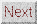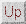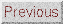Next: Bandpower Estimation Up: DATA ANALYSIS Previous: DATA ANALYSIS
Wayne Hu 2001-10-15